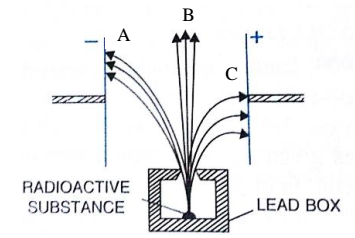# ICSE Class 10 Physics 2018 Question Paper

Section I is compulsory. You have to attempt any four questions from Section II. There are total 10 questions.

### Section I (40 Marks)

Attempt all questions from this Section

Question 1

(a) (i) State and define the S.I. unit of power.
(ii) How is the unit horse power related to the S.I. unit of power? 

(b) State the energy changes in the following cases while in use:
(i) An electric iron.
(ii) A ceiling fan. 

(c) The diagram below shows a lever in use: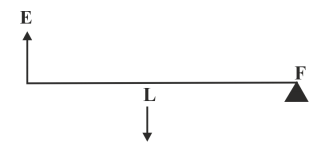(i) To which class of levers does it belong?
(ii) Without changing the dimensions of the lever, if the load is shifted towards the fulcrum what happens to the mechanical advantage of the lever? 

(d) (i) Why is the ratio of the velocities of light of wavelengths 4000 Å and 8000 Å in vacuum 1:1?
(ii) Which of the above wavelengths has a higher frequency? 

(e) (i) Why is the motion of a body moving with a constant speed around a circular path said to be accelerated?
(ii) Name the unit of physical quantity obtained by the formula 2 𝐾/𝑉2.
Where K: kinetic energy, V: Linear velocity. 

Question 2

(a) The power of a lens is –5D.
(i) Find its focal length.
(ii) Name the type of lens. 

(b) State the position of the object in front of a converging lens if:
(i) It produces a real and same size image of the object.
(ii) It is used as a magnifying lens. 

(c) (i) State the relation between the critical angle and the absolute refractive index of a medium.
(ii) Which colour of light has a higher critical angle? Red light or Green light. 

(d) (i) Define scattering.
(ii) The smoke from a fire looks white. Which of the following statements is true?
1. Molecules of the smoke are bigger than the wavelength of light.
2. Molecules of the smoke are smaller than the wavelength of light. 

(e) The following diagram shows a 60°, 30°, 90° glass prism of critical angle 42°. Copy the diagram and complete the path of incident ray AB emerging out of the prism marking the angle of incidence on each surface.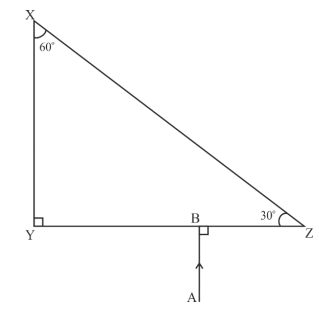Question 3

(a) Displacement distance graph of two sound waves A and B, travelling in a medium, are as shown in the diagram below.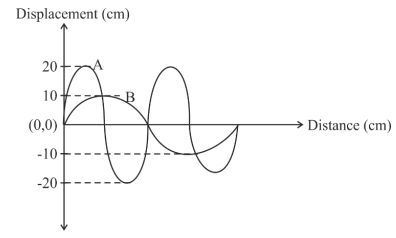Study the two sound waves and compare their:
(i) Amplitudes
(ii) Wavelengths 

(b) You have three resistors of values 2Ω, 3Ω and 5Ω. How will you join them so that the total resistance is more than 7Ω?
(i) Draw a diagram for the arrangement.
(ii) Calculate the equivalent resistance. 

(c) (i) What do you understand by the term nuclear fusion?
(ii) Nuclear power plants use nuclear fission reaction to produce electricity. What is the advantage of producing electricity by fusion reaction? 

(d) (i) What do you understand by free vibrations of a body?
(ii) Why does the amplitude of a vibrating body continuously decrease during damped vibrations? 

(e) (i) How is the e.m.f. across primary and secondary coils of a transformer related with the number of turns of coil in them?
(ii) On which type of current do transformers work? 

Question 4

(a) (i) How can a temperature in degree Celsius be converted into S.I. unit of temperature?
(ii) A liquid X has the maximum specific heat capacity and is used as a coolant in Car radiators. Name the liquid X. 

(b) A solid metal weighing 150 g melts at its melting point of 800°C by providing heat at the rate of 100 W. The time taken for it to completely melt at the same temperature is 4 min. What is the specific latent heat of fusion of the metal? 

(c) Identify the following wires used in a household circuit:
(i) The wire is also called as the phase wire.
(ii) The wire is connected to the top terminal of a three pin socket. 

(d) (i) What are isobars?
(ii) Give one example of isobars. 

(e) State any two advantages of electromagnets over permanent magnets. 

### Section II (40 Marks)

Attempt any four questions from this Section

Question 5

(a) (i) Derive a relationship between S.I. and C.G.S. unit of work.
(ii) A force acts on a body and displaces it by a distance S in a direction at an angle θ with the direction of force. What should be the value of θ to get the maximum positive work? 

(b) A half metre rod is pivoted at the centre with two weights of 20 gf and 12 gf suspended at a perpendicular distance of 6 cm and 10 cm from the pivot respectively as shown below.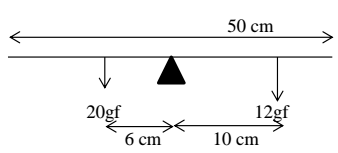(i) Which of the two forces acting on the rigid rod causes clockwise moment?
(ii) Is the rod in equilibrium?
(iii) The direction of 20 gf force is reversed. What is the magnitude of the resultant moment of the forces on the rod? 

(c) (i) Draw a diagram to show a block and tackle pulley system having a velocity ratio of 3 marking the direction of load (L), effort (E) and tension (T).
(ii) The pulley system drawn lifts a load of 150 N when an effort of 60 N is applied. Find its mechanical advantage.
(iii) Is the above pulley system an ideal machine or not? 

Question 6

(a) A ray of light XY passes through a right angled isosceles prism as shown below.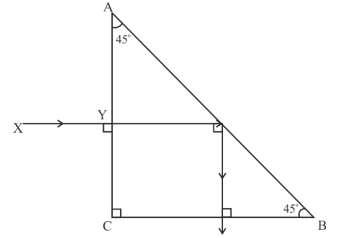(i) What is the angle through which the incident ray deviates and emerges out of the prism?
(ii) Name the instrument where this action of prism is put into use.
(iii) Which prism surface will behave as a mirror? 

(b) An object AB is placed between O and F1 on the principal axis of a converging lens as shown in the diagram.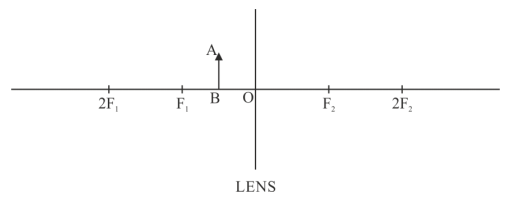Copy the diagram and by using three standard rays starting from point A, obtain an image of the object AB. 

(c) An object is placed at a distance of 12 cm from a convex lens of focal length 8 cm. Find:
(i) the position of the image
(ii) nature of the image 

Question 7

(a) Draw the diagram of a right angled isosceles prism which is used to make an inverted image erect. 

(b)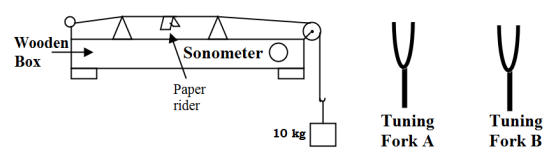The diagram above shows a wire stretched over a sonometer. Stems of two vibrating tuning forks A and B are touched to the wooden box of the sonometer. It is observed that the paper rider (a small piece of paper folded at the centre) present on the wire flies off when the stem of vibrating tuning fork B is touched to the wooden box but the paper just vibrates when the stem of vibrating tuning fork A is touched to the wooden box.

(i) Name the phenomenon when the paper rider just vibrates.
(ii) Name the phenomenon when the paper rider flies off.
(iii) Why does the paper rider fly off when the stem of tuning fork B is touched to the box? 

(c) A person is standing at the sea shore. An observer on the ship which is anchored in between a vertical cliff and the person on the shore, fires a gun. The person on the shore hears two sounds, 2 seconds and 3 seconds after seeing the smoke of the fired gun. If the speed of sound in the air is 320 ms-1 then calculate:
(i) the distance between the observer on the ship and the person on the shore.
(ii) the distance between the cliff and the observer on the ship.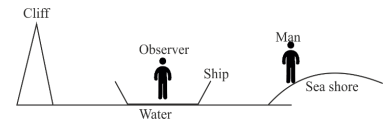Question 8

(a) (i) A fuse is rated 8A. Can it be used with an electrical appliance rated 5 KW, 200 V? Give a reason.
(ii) Name two safety devices which are connected to the live wire of a household electric circuit. 

(b) (i) Find the equivalent resistance between A and B.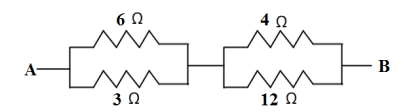(ii) State whether the resistivity of a wire changes with the change in the thickness of the wire. 

(c) An electric iron is rated 220V, 2kW.
(i) If the iron is used for 2h daily find the cost of running it for one week if it costs Rs 4.25 per kWh.
(ii) Why is the fuse absolutely necessary in a power circuit? 

Question 9

(a) (i) Heat supplied to a solid changes it into liquid. What is this change in phase called?
(ii) During the phase change does the average kinetic energy of the molecules of the substance increase?
(iii) What is the energy absorbed during the phase change called? 

(b) (i) State two differences between “Heat Capacity” and “Specific Heat Capacity”.
(ii) Give a mathematical relation between Heat Capacity and Specific Heat Capacity. 

(c) The temperature of 170 g of water at 50°C is lowered to 5°C by adding certain amount of ice to it. Find the mass of ice added.
Given: Specific heat capacity of water = 4200 J kg-1 ⁰C-1 and Specific latent heat of ice = 336000 J kg-1 

Question 10

(a)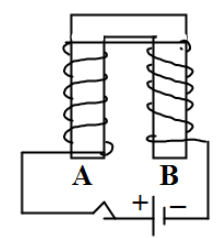The diagram shows a coil wound around a U shape soft iron bar AB.
(i) What is the polarity induced at the ends A and B when the switch is pressed?
(ii) Suggest one way to strengthen the magnetic field in the electromagnet.
(iii) What will be the polarities at A & B if the direction of current is reversed in the circuit? 

(b) The ore of Uranium found in nature contains 92U238 and 92U235. Although both the isotopes are fissionable, it is found out experimentally that one of the two isotopes is more easily fissionable.
(i) Name the isotope of Uranium which is easily fissionable.